# Impedance Matching: Formula, Circuit & Applications

Contents

## What is Impedance Matching?

Impedance matching is defined as the process of designing the input impedance and output impedance of an electrical load to minimize the signal reflection or maximize the power transfer of the load.

An electrical circuit consists of power sources like amplifier or generator and electrical load like a light bulb or transmission line have a source impedance. This source impedance is equivalent to resistance in series with reactance.

According to the maximum power transfer theorem, when the load resistance is equal to the source resistance and load reactance is equal to negative of the source reactance, the maximum power is transferred from source and load. It means that the maximum power can be transfer if the load impedance is equal to the complex conjugate of the source impedance.

In the case of the DC circuit, the frequency is not considered. Hence, the condition is satisfied if the load resistance equals the source resistance. In the case of the AC circuit, the reactance depends on the frequency. Hence, if the impedance is matched for one frequency may not match if the frequency is changed.

## Smith Chart Impedance Matching

The smith chart was invented by Philip H Smith and T. Mizuhashi. It is a graphical calculator used for solving complex problems of transmission lines and matching circuits. This method is also used to display the behavior of the RF parameter at one or more frequencies.

Smith chart used to display parameters like impedances, admittances, noise figure circles, scattering parameters, reflection coefficient, and mechanical vibrations, etc. Hence, most RF analysis software includes a smith chart to display as it is one of the most important methods for RF engineers.

There are three types of smith charts;

• Impedance Smith Charts (Z Charts)
• Admittance Smith Charts (Y Charts)
• Immittance Smith Charts (YZ Charts)

## Impedance Matching Circuit and Formula

For a given load resistance R, we will find a circuit that matches the driving resistance R’ at frequency ω0. And we design L matching circuit (as shown in below figure).

Let’s find the admittance (Yin) of the above circuit.

Consider that, the Resistor (R) and Inductor (L) are in series. And this combination is in parallel with the Capacitor (C). Hence, the Impedance is,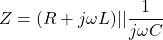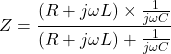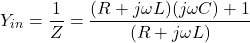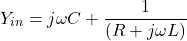Now, we separate the imaginary part and real part of the above equation with the help of complex conjugate.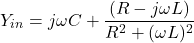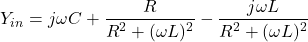Now,

(1)At ω = ω0, we have resistance for Yin, which should be set to R’.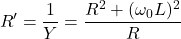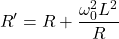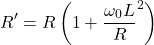(2)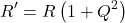Here, Q is the Q-factor, for series L and R network, Q-factor is equal to,

(3)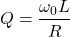Steps to design this circiut.

Step-1 For given R and R’, find the required Q from eq-2.
Step-2 For given ω0, Find the required from eq-3.
Step-3 From eq-1, find the required Y to give the selected resonant frequency.

## Why is Impedance Matching Important

The impedance matching is most important in the case of the high speed and high-frequency devices. When you designing a PCB for such type of application, you must keep in mind to match the impedance of source and load.

In ultra-high frequency applications, the impedance matching is a very difficult task for the design engineers. It is also challenging in designing RF and microwave circuits. The impact of a small error in impedance matching results in pulse distortion and reflection of signals.

As the frequency increases the window of error is decreases. And for higher frequencies the importance of maximum power transfer becomes critical. The circuit works properly and efficiently if the impedance is matched perfectly. If the impedance is not matched correctly, there are many negative effects in the circuit because of the reflection of signals.

These reflected waves matched with the transmitted signals. And it’s may cause delays in data, phase distortion, and reduce the ratio of signal to noise.

## Impedance Matching Applications

In electrical and electronic circuit design, the main goal of a designer is to achieve maximum power that can deliver from the source to load. In almost all applications, impedance matching is necessary.

We have discussed a few applications of impedance matching.

### Impedance Matching Transformer

The transformer is used to match the impedance of source and load. The input power of the transformer is the same as the output power of the transformer. It is only changing the voltage level of electrical energy. It does not change the power level.

The turns ratio is set according to match the impedance of both sides. The number of turns of low voltage winding is less. And hence the impedance of the low voltage winding is less compared to the impedance of high voltage winding.

Therefore, to match the impedance, the transformer is used to connect the source and load by choosing an appropriate number of turns of windings. Sometimes, this transformer is also known as a matching transformer.

The turns ratio of the matching transformer is defined as the square root of the ratio of source resistance to the load resistance.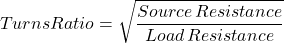### Antenna Impedance Matching

The common application of Impedance matching is in coupling an antenna with television. The antenna is a source because it provides the signal. And the television is considered a load because it receives the signal.

For example, the resistance of antenna and cable is 75 ohms and the resistance of television is 300 ohms. In this condition, because of different impedance, the maximum power cannot be transferred and results in poor signal reception.

Now, to avoid this condition, we use a transformer to match the impedance of the antenna and television. The turns ratio of the transformer is calculated the same as the above equation.

Now for this example, if we put the value of source impedance and load impedance, it can be written as,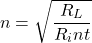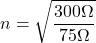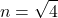From the above equation, the turns ratio is 1:2. And the transformer is connected as shown in the below figure.

### Transmission Line Impedance Matching

The transmission line is used to transfer electrical energy from source to load. It is very important that the energy loss occurs during the power transfer is zero or as minimum as possible. To accomplish this task, the source and load impedances have to match the characteristic impedance of the transmission line.

The characteristic impedance is a ratio of the voltage and current wave at any point on the transmission line. For a long transmission line, it is possible to have different characteristic impedance at different positions of a transmission line.

If the impedance is not matched, the signal reached the load and reflect back to the source. It will produce a standing wave. The amount of reflected power can measure by the reflection coefficient. And the equation of reflection coefficient is,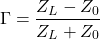Where,
ZL = Line Impedance
Z0 = Characteristic Impedance

The ideal value of the reflection coefficient is zero. In this condition, the load impedance is the same as the characteristic impedance. But in a practical transmission line, the value of the reflection coefficient is kept as small as possible.

### Audio / Headphone Impedance Matching

In this example, the source is the device in which the headphone is connected and the headphone is considered as the load. To achieve the highest audio quality, the source impedance and load impedance should be matched.

The matched impedance ensures the maximum power can transfer from the audio source to the headphone.

For portable devices, low impedance headphones are designed to work properly with adequate sound quality. The high impedance headphones are designed for robust amplification to perform their best result. And generally, high impedance headphones are older or professional studio-specific designs.

Want To Learn Faster? 🎓
Get electrical articles delivered to your inbox every week.
No credit card required—it’s 100% free.